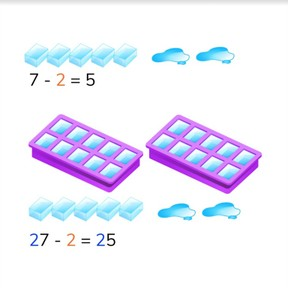Subtraction to 100 via simplification

# Subtraction to 100 via simplification

No account needed.8,000 schools use Gynzy92,000 teachers use Gynzy1,600,000 students use Gynzy

## General

Students learn that they can split a subtraction problem into tens and ones and use the subtraction problem with ones as a first step to subtract with tens.

2.NBT.B.5
2.OA.A.1

## Relevance

It is important to learn this to help make subtraction easier and faster to do.

## Introduction

Start with a few counting exercises on the interactive whiteboard to 100. Have students count quantities and then explain their strategies for counting. It is possible to skip count in 2s, 3s, 5s or one by one. Then do a quick pop quiz with subtraction problems to 20. How many can students get correct in two minutes?

## Development

Show students a set of subtraction problems that use simplification. Ask students if they notice anything about the subtraction problems. They should notice that the difference between the two problems is 10. Namely that 10 is added to the first number (minuend) and to the difference. These subtraction problems are related. Tell students that you can sometimes solve a subtraction problem with tens faster if you first solve the subtraction problem without the tens. You can use that difference as a first step. If you know 7 - 2 = 5, then you can quickly determine that 27 - 2 = 25. Practice this with the ice cubes on the interactive whiteboard. Emphasize that they don't need to recalculate the subtraction problem, because they can use the first step they already solved. 20 is added to the subtraction problem, so 20 is also added to the difference. Check if students are able to solve a subtraction problem without blocks on the interactive whiteboard as well by using the example of colored pencils. First with 9 - 4, then with 19 - 4, and then with 29 - 4. Show them a grid of 100 and tell them that they can use this as support for solving this kind of subtraction problem. If you go back, you take one away. If you go down, you add 10. Do a few more subtraction problems with the students, but without visual support. They can imagine the MAB blocks in their head to help imagine the tens that are added to the second step of the subtraction problem.

Check that students understand subtraction to 100 using simplification by asking the following questions:
- 7 - 2 = 5, That means 17 - 2 = ?
- 14 - 3 = 11. 54 - 3 = 51. How do you know this is true?
- 18 - 9 = 9 Which subtraction problem(s) can you now easily solve?

## Guided practice

Students first are given subtraction problems in which the subtraction problem with ones is given and they must solve with tens. Then they must solve the subtraction problems with tens and ones. Finally they are given subtraction problems including regrouping.

## Closing

Repeat why it is important to learn how to subtract using simplification. It makes subtraction easier and faster. If you know the subtraction problem of the ones, you can easily solve the subtraction problem with the tens. Check that students have understood by doing two subtraction problems as a class, one with visual support and one without.

## Teaching tips

Students who have difficulty with this can make use of MAB blocks or money. Give them a 5 dollar bill and a 1 dollar bill. They know that 5 - 1 = 4. If you now give them a 10 dollar bill, the problem becomes 15 - 1 = 14. If the get another 10 dollar bill the problem becomes 25 - 1. Repeat this with other numbers, Emphasize that they don't need to recalculate the difference, but that this is a first step to solving the subtraction problem with tens.

## Instruction materials

optional: Money, or MAB blocks/manipulatives

### The online teaching platform for interactive whiteboards and displays in schools

• Save time building lessons

• Manage the classroom more efficiently

• Increase student engagement